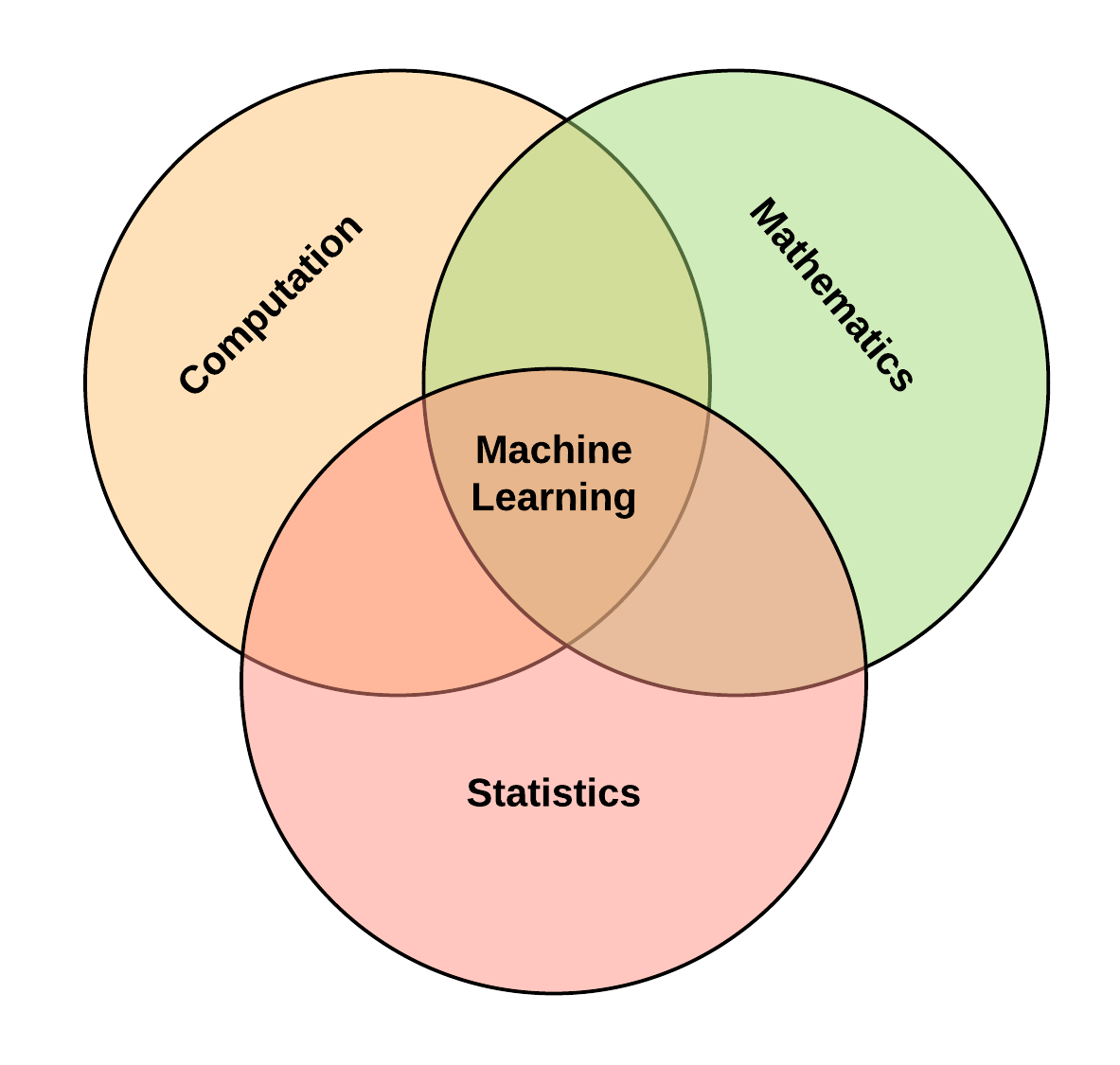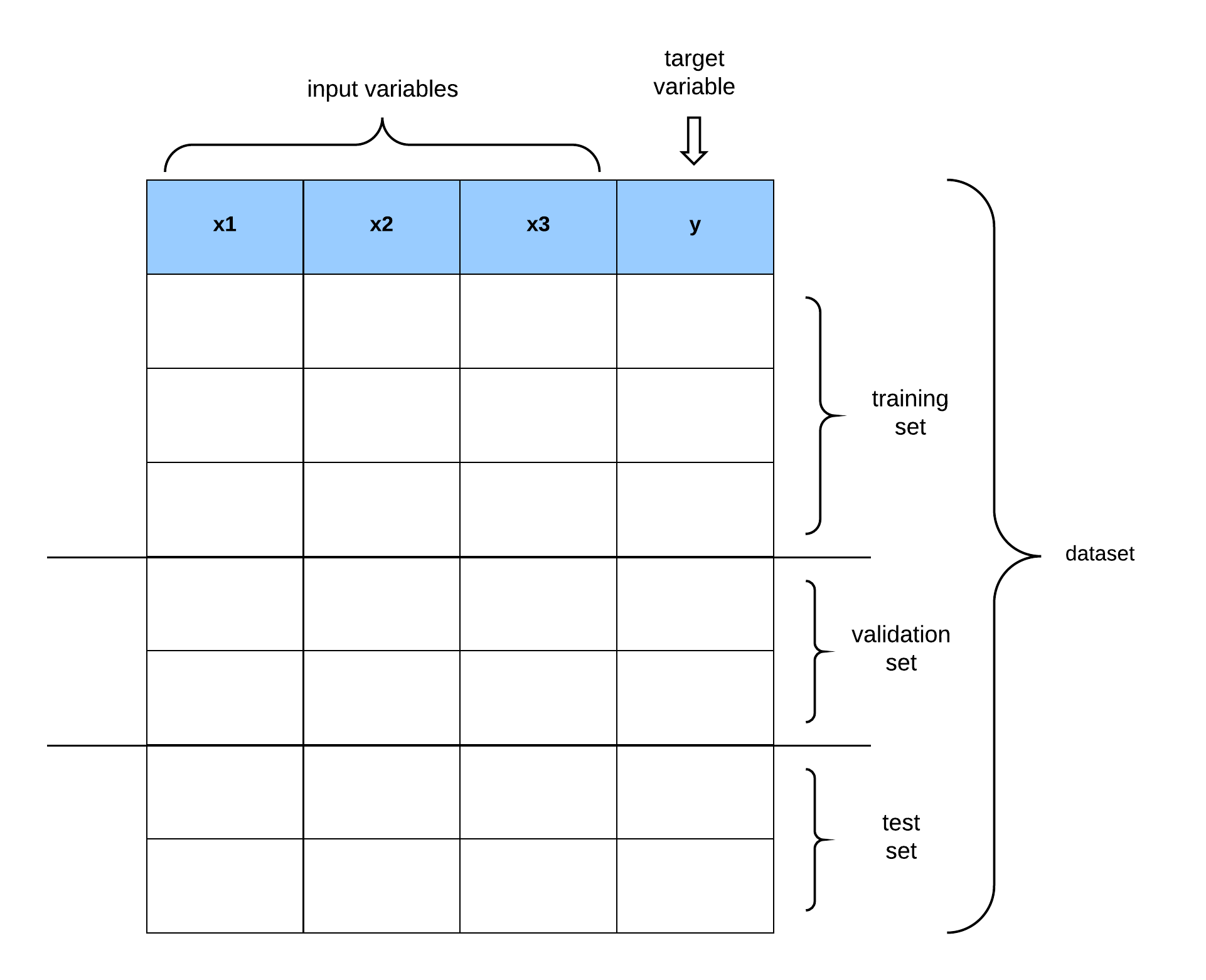Machine learning is a system of computing tools, and scientific methods for training a computer to learn and make inferences from data. It is a sub-field of Artificial Intelligence that aims to mimic the human biological approach to learning by receiving signals from the environment in the form of data and processing that data to gain intelligence on the state of the world.

Moreso, Machine learning is an interdisciplinary study, although a lot of research advances is in the domain of computer science, the field is built on the foundations of mathematics, statistics, cognitive sciences and other areas such as psychology, and linguistics.Figure 1: Foundations of ML

### The Role of Data

At the core of machine learning is data. Data is key to the current evolution and further advancement of this field. Data serves as the natural representation of the environment. Just as it is for humans (the ultimate form of intelligence), so it is for a machine, learning is simply inconceivable without data.

However, unlike humans that have free and unfettered access to an unlimited repertoire of extremely rich data, the case is not the same for machines. But in the early 1990s, the internet was born, and by the dawn of the century, it became a super-highway for data distribution. This data explosion paved the way for research, development and rapid prototyping of machine learning algorithms and techniques in various domains. The internet made data ubiquitous and has in turn improved the performance and sophistication of machine learning algorithms.

### Core Components of Machine Learning

The three distinct blocks that characterize machine learning are:

1. Supervised learning
2. Unsupervised learning
3. Reinforcement learning

Let’s look briefly at each block.

1. Supervised learning: The idea behind supervised learning is to teach the computer to learn from labeled data. The computer learns the patterns/ relationships between the predictor attributes and the output variable.

2. Unsupervised learning: In the case of unsupervised learning, there is no such guidance with labeled examples, rather the computer attempts to determine the unknown structure of the data.

3. Reinforcement learning: The concept of reward and penalty is central to reinforcement learning. Here the learner interacts with a non-stationary environment. The machine is rewarded when the response from the environment is favorable and penalized when the response is unfavorable.

### Training, Test and Validation datasets

We can misjudge the performance of our learning models if we evaluate the model performance with the same samples used to train the model as explained in the previous sub-section.

To properly evaluate the performance of a learning algorithm, we need to set aside some data for testing purposes. This hold-out data is called a test set. Another situation arises when we have trained the model on a dataset, and we need to improve the performance of the model by tuning some of the learning algorithm’s hyperparameters. Which dataset will we use to train the adjusted model?

We cannot use the test set for model tuning because if we do that, the model learns the test data set and consequently renders it unusable for model evaluation. Thus, we divide the data set into three compartments namely:

1. the training set (to train the model),
2. the validation set (for model tuning), and
3. the test set (for model evaluation)

A common and straightforward strategy is to split 60% of the dataset for training, 20% for validation, and the final 20% for testing. This strategy is popularly known as the 60/20/20 rule. We will discuss more sophisticated methods for resampling (i.e., using subsets of available data) for machine learning.Figure 2: Training, test and validation set

The concept of bias vs. variance is very central to machine learning and is key to understanding how the model is performing, as well as in deciding how best to improve the model.

Succinctly defined, bias is when the model oversimplifies the learning problem or when the model fails to accurately capture the complex relationships inherent in the dataset, thereby making the model unable to generalize to new examples.

Variance, on the other hand, is when the model learns too closely the intricate patterns of the dataset and in the process learns the irreducible noise inherent in every data set. When the learning algorithm entirely learns the patterns, including the noise of the training data, it fails to generalize when exposed to unseen data.Figure 3: Bias and variance

### Evaluating Model Quality

Evaluation metrics give us a way to know how well our model is doing or performing. We evaluate the training data to get the training set accuracy, while we evaluate using test data to know the accuracy on unseen examples. Evaluation of test data helps us to know if our models are high performing, or if they are suffering from high bias or variance.

The learning problem determines the type of evaluation metric to use. Typically, for real-valued prediction problems, we use the mean-squared error (MSE) for evaluation. Whereas, for “categorical” classification problems, it is best to plot a confusion matrix to get a clear picture of how many samples are correctly classified or misclassified. From the confusion matrix, one can know other useful metrics for evaluating classification problems such as accuracy, precision, recall, and F1.

### Resampling Techniques

This section describes another important concept for evaluating the performance of a supervised learning algorithm and for fine-tuning the learning algorithm (i.e., adjusting the parameters of a learning algorithm for better results). Resampling methods are a set of techniques that involves selecting a subset of the available dataset, training on that data subset and using the remainder of the data to evaluate the trained model.

This process involves creating subsets of the available data into a training set and a validation set. The training set is used to train the model while the validation set will evaluate the performance of the learned model on unseen data. Typically, this process will be carried out repeatedly for a set number of times to get a statistical approximation of the training and test error measures. Examples of resampling techniques are LOOCV and k-fold cross-validation. Remember, we are most interested in the test error rate, as this gives us a reasonable estimate on the ability of the model to generalize to new examples.

### Machine learning algorithms

Three broad groups of machine learning algorithms are:

1. Linear methods (parametric methods)
2. Non-linear methods (non-parametric methods)
3. Ensemble methods

Note: Some algorithms can turn up in multiple groups.

1. Linear methods assumes a parametric form of the underlying structure of the data. Examples of linear algorithms are:
• Linear regression,
• Logistic regression,
• Support vector machines
2. Non-Linear methods do not assume a structural form of the dataset. It uses the examples to learn the internal representation of the data. Examples of non-linear algorithms are:
• K-Nearest Neighbors,
• Classification & regression trees,
• Support vector machines,
• Neural networks
3. Ensemble methods combine the output of multiple algorithms to build a better model estimator, that is, it combines algorithms to create a model that generalizes better to unseen examples. Two major classes of ensemble methods are:
• Boosting, and
• Bagging

### Machine Learning Packages

Machine learning has made a significant transition from the mathematical domain to the software engineering arena over the past ten years. An evidence of this shift is the number of machine learning packages now available as reusable software components for easy implementation in projects. Packages hide the underlying gory mathematical details from the user.

Popular machine learning packages are:

• sickit-learn (Python programming language)
• caret (R programming language)
• Weka (Java programming language)
• dlib (C++ programming language)

The entirety of machine learning builds on the key points discussed in this post. Hopefully, this demystifies the field well enough to engage in discussions on machine learning and in extension artificial intelligence.